Vibroengineering PROCEDIA

Published: 07 June 2019

# Misfire fault diagnosis of diesel engine based on VMD and XWT

Jide Jia1
Gang Ren2
Jianmin Mei3
1School of Mechanical and Manufacturing Engineering, Xiamen Institute of Technology, Xiamen, 361024, China
2Automobile NCO School, Army Military Transportation University, Bengbu, 233011, China
3, 1Projection Equipment Support Department, Army Transportation University, Tianjin, China
Corresponding Author:
Gang Ren
Views 80

#### Abstract

For diesel engine misfire fault diagnosis under strong noise, a new method based on variational mode decomposition (VMD) and cross wavelet transform (XWT) is proposed. Firstly, the vibration signal of cylinder head is processed by stages according to the working cycle of diesel engine, and then the vibration signal of each working cycle is re-sampled according to the same angle. Then the vibration signal of cylinder head is decomposed by VMD to denoise adaptively and reconstruct the signal. Next, XWT is used to analyze the time-frequency correlation of any two continuous working cycle signals, and the non-periodic components and random noise in the vibration signals are further eliminated to extract the combustion characteristics of diesel engines. Finally, the misfire fault of diesel engine is diagnosed by calculating the energy proportion of each cylinder in time-frequency space. The effectiveness of the proposed method is verified by simulation and experiment.

## 1. Introduction

There are many factors affecting the combustion of diesel engine and the working process is complex, which results in the multi-component and non-stationary characteristics of the signal. It is difficult to monitor and diagnose the combustion state of diesel engine. Domestic and foreign scholars have done a lot of research on diesel engine misfire fault, and put forward a variety of diagnostic methods, such as cylinder pressure diagnostic method , instantaneous speed diagnostic method , exhaust noise diagnostic method, and cylinder head vibration and sound diagnostic method . However, no matter what method is used, feature extraction is one of the key steps in the diagnosis process. Whether the extracted feature information can characterize the combustion characteristics of diesel engine is directly related to the accuracy of diagnosis results.

Empirical mode decomposition (EMD) is used to extract eigenvalues, which can reconstruct signals and denoise. However, EMD has the problems of endpoint effect and modal aliasing. Although a series of improvements have been made, such as EEMD, CEEMDAN, endpoint effect is still unavoidable, as well as the problems of large amount of computation and poor anti-noise ability. Wavelet transform (WT) is used to extract eigenvalues, which has been widely used in the field of diesel engine fault diagnosis. However, WT can only analyze a single time series signal, and it is difficult to analyze the interaction and time-frequency correlation between two time series.

In recent years, Konstantin Dragomiretskiy  proposed a new variable adaptive decomposition method, Variational Mode Decomposition (VMD). The method is essentially composed of a plurality of adaptive Wiener filter and has good noise robustness. Compared with EMD, VMD has strong mathematical theory basis. At the same time, VMD rejects the method of recursive screening stripping. So VMD can effectively alleviate or avoid a series of problems which appear in EMD and has high operation efficiency of decomposition . VMD has been fully utilized in the field of engineering.

Cross-wavelet transform (XWT) is a new signal analysis technology based on traditional wavelet transform. Through the combination of wavelet transform and cross-spectrum analysis, the relationship between two time series in time-frequency domain can be studied from the perspective of multi-time scale. At the same time, the different performance of signal and noise in time-frequency correlation is utilized to achieve the purpose of denoising and signal enhancement .

After a lot of research, a new diagnosis method for diesel engine misfire fault based on VMD and XWT is presented in this paper. The proposed method is applied to diesel engine misfire fault diagnosis. The fault feature is successfully extracted, and diesel engine misfire fault can be diagnosed effectively.

## 2. Feature extraction based on VMD and XWT

### 2.1. VMD

Detailed algorithms for VMD can be found in reference . The variational constraint problem can be defined as follows:

1

where ${u}_{k}$ is the modal component, ${w}_{k}$ is the central frequency for the modal component, $\delta \left(t\right)$ is the unit pulse function, and * is the convolution symbol.

In the VMD algorithm, the secondary penalty factor and the Lagrangian multiplication operator are used. Then, the alternating direction method is introduced. ${u}_{k}^{n+1}$, ${\omega }_{k}^{n+1}$, and are constantly updated.

The expression for the modal component ${u}_{k}^{n+1}$ in frequency domain is:

2
${\stackrel{^}{u}}_{k}^{n+1}\left(\omega \right)=\left(\stackrel{^}{f}\left(\omega \right)-{\sum }_{i}{\stackrel{^}{u}}_{i}\left(\omega \right)+\frac{\stackrel{^}{\lambda }\left(\omega \right)}{2}\right)\frac{1}{1+2\alpha \left(\omega -{\omega }_{k}{\right)}^{2}}$

where is the center of the modal component power spectrum. The Wiener filter is introduced, which makes the VMD algorithm have better noise robustness.

Similarly, the expression for the central frequency ${\omega }_{k}^{n+1}$:

3
${\omega }_{k}^{n+1}\left(\omega \right)=\frac{{{\int }_{0}^{\infty }\omega \left|{\stackrel{^}{u}}_{k}\left(\omega \right)\right|}^{2}d\omega }{{{\int }_{0}^{\infty }\left|{\stackrel{^}{u}}_{k}\left(\omega \right)\right|}^{2}d\omega }.$

The stopping condition of the iteration is:

4
$\frac{{{\sum }_{k=1}^{K}‖{{\stackrel{^}{u}}_{k}}^{n+1}-{{\stackrel{^}{u}}_{k}}^{n}‖}_{2}^{2}}{{‖{{\stackrel{^}{u}}_{k}}^{n}‖}_{2}^{2}}

The VMD algorithm is a linear transformation, so the signal can be reconstructed. The reconstructed signal can be represented as:

5
$\stackrel{^}{f}\left(t\right)={\sum }_{k=1}^{K}{\stackrel{^}{u}}_{k},$

where ${\stackrel{^}{u}}_{k}$ is the final modal component, after the iteration is stopped.

### 2.2. XWT

The continuous wavelet transform of the signal $x\left(t\right)$ is:

6
${W}_{x}\left(a,b\right)={\int }_{-\mathrm{\infty }}^{\mathrm{\infty }}x\left(t\right){\psi }_{a,b}^{\mathrm{*}}\left(t\right)d\left(t\right)=〈x\left(t\right),{\psi }_{a,b}\left(t\right)〉,$
7
${\psi }_{a,b}\left(t\right)={\left|a\right|}^{-\frac{1}{2}}\psi \left(\frac{t-b}{a}\right),$

where $a$ is the scale factor and $b$ is the translation factor; ${\psi }_{a,b}\left(t\right)$ is a wavelet basis function, and * denotes complex conjugation.

Wavelet transform expands one-dimensional signal in time-frequency domain and can analyze any details of signal change. For any two signals $x\left(t\right)$ and $y\left(t\right)$, the XWT of two signals established on the basis of wavelet transform can be expressed as :

8
${C}_{x,y}\left(a,b\right)={W}_{x}\left(a,b\right){{W}_{y}}^{\mathrm{*}}\left(a,b\right).$

The coefficients of XWT represent the correlation of two signals with wavelet coefficients at the same scale. XWT can analyze the correlation between the two signals in both time and frequency domains. It reflects the correlation between the two signals in the time-frequency domain. The larger the correlation, the closer the correlation between the two signals .

Morlet wavelet is chosen as the wavelet basis function in XWT analysis, which has good localization properties in both time and frequency domains. The expressions in time domain and frequency domain are as follows:

9
$\psi \left(t\right)={\pi }^{-\frac{1}{4}}\mathrm{e}\mathrm{x}\mathrm{p}\left(j{\omega }_{0}t\right)\mathrm{e}\mathrm{x}\mathrm{p}\left(-\frac{{t}^{2}}{2}\right),$
10
$\stackrel{^}{\psi }\left(a\omega \right)={\pi }^{\frac{1}{4}}\mathrm{e}\mathrm{x}\mathrm{p}\left[\frac{-{\left(a\omega -{\omega }_{0}\right)}^{2}}{2}\right],$

where the value of ${\omega }_{0}$ is 6 to ensure good local performance in time-frequency distribution.

### 2.3. Proposed method

Firstly, the vibration signal of cylinder head is re-sampled in segments and equal angles. Then the VMD is used to decompose and reconstruct the cylinder head vibration signal, and XWT is used to analyze the time-frequency correlation. Finally, according to the energy of each cylinder in the time-frequency correlation spectrum, the misfire diagnosis of diesel engine is carried out.

## 3. Experimental results

### 3.1. Simulation

To verify the proposed method, a multi-component simulation signal consisting of four AM-FM components and noise is established as follows:

11
$S={s}_{1}+{s}_{2}+{s}_{3}+{s}_{4}+n\left(t\right),$
12
${s}_{1}=\mathrm{e}\mathrm{x}\mathrm{p}\left(-at\right)\left(\mathrm{s}\mathrm{i}\mathrm{n}2\pi {f}_{1}{k}_{1}{t}_{1}\right),$
13
${s}_{2}=\mathrm{e}\mathrm{x}\mathrm{p}\left(-at\right)\left(\mathrm{s}\mathrm{i}\mathrm{n}2\pi {f}_{2}{k}_{2}{t}_{2}\right),$
14
${s}_{3}=\mathrm{e}\mathrm{x}\mathrm{p}\left(-at\right)\left(\mathrm{s}\mathrm{i}\mathrm{n}2\pi {f}_{3}{k}_{3}{t}_{3}\right),$
15
${s}_{4}=\mathrm{e}\mathrm{x}\mathrm{p}\left(-at\right)\left(\mathrm{s}\mathrm{i}\mathrm{n}2\pi {f}_{4}{k}_{4}{t}_{4}\right),$

where $t=mod\left(kdt,1/{f}_{m}\right)$; $k$ is a natural number; $dt=$ 5×10-5; ${f}_{m}=$ 10 Hz; $a=$ 1800 Hz; ${k}_{1}=$ 1:250; ${k}_{2}=$ 1:250; ${k}_{3}=$ 1:250; ${k}_{4}=$ 1:150; ${f}_{1}=$ 1 kHz; ${f}_{2}=$ 3 kHz; ${f}_{3}=$ 5 kHz; ${f}_{4}=$ 7 kHz. The signal sampling frequency is 1500Hz, and the sampling number is 2000 Hz.

WT, VMD and VMD+XWT are used to analyze the time-frequency of the simulation signal, and the time-frequency analysis results are shown in Fig. 1.

Fig. 1The time-frequency diagram of simulation signal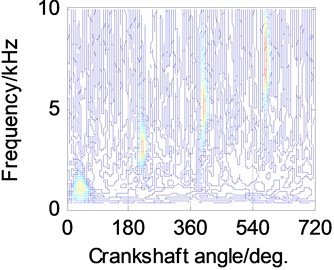a)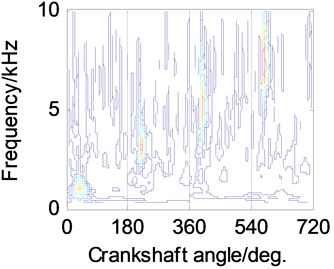b)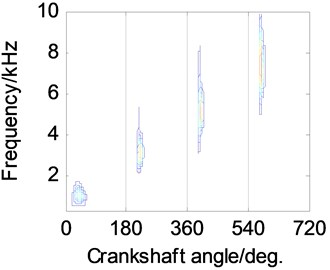c)

The change of contour nephogram corresponds to the change of signal energy in time-frequency space. The light-colored area corresponds to the higher intensity energy value, while the dark-colored area corresponds to the lower intensity energy value. Other time-frequency diagrams in this paper show the same. From Fig. 1(a), it can be seen that WT has insufficient noise attenuation for simulation signal. From Fig. 1(b), it can be seen that VMD has obvious attenuation for simulation signal noise. From Fig. 1(c), it can be seen that the method based on VMD and XWT has a great attenuation to the simulation signal noise. By combining VMD and XWT, the main components of the simulation signal are extracted completely, and the interference noise is basically eliminated.

### 3.2. Experiment condition

The vibration signal of engine cylinder head contains abundant information, which can effectively reflect the change of combustion state of diesel engine. The cylinder head vibration signal can be used to monitor and diagnose the combustion state of diesel engine. The measurement method is reliable and can realize on-line monitoring and diagnosis. The test object is HJ493 diesel engine.

A 601A01 ICP vibration sensor is installed on the cylinder head between the second cylinder and the third cylinder of the diesel engine. QSY8104 external card type high-pressure tubing pressure sensor is clamped on the first cylinder high-pressure tubing of diesel engine. The speed of the engine is measured by the oil pressure pulse signal of the high-pressure tubing and the position of the first cylinder stop point is measured. The signal sampling frequency is 12.8 kHz and the data sample length is 12288. Five working conditions of diesel engine are simulated by controlling the fuel supply of each cylinder injector: normal combustion state of diesel engine, poor combustion of the first cylinder, poor combustion of the second cylinder, poor combustion of the third cylinder and poor combustion of the fourth cylinder. Vibration signals of cylinder head surface were collected at 700 r/min of diesel engine speed under normal and abnormal conditions.

### 3.3. Experimental data processing

According to the proposed method, the vibration signals of cylinder head in five states are processed respectively. The effects of aperiodic component and random noise are effectively attenuated, and the combustion state characteristics are enhanced. The results are shown in Fig. 2.

Fig. 2Three-dimensional time-frequency diagram of vibration signals in different combustion states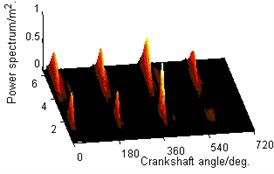a)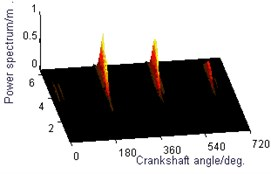b)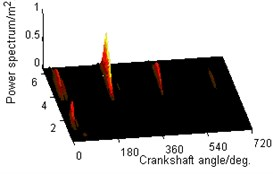c)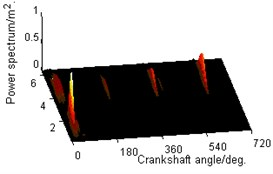d)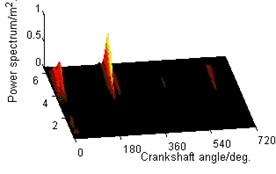e)

As can be seen from Fig. 2, when the diesel engine is in normal combustion state, the combustion state of the four cylinders is basically the same. The energy of each cylinder is different, but the difference is not significant. When a cylinder is misfired, the combustion balance of the whole diesel engine is destroyed, and the energy of the misfired cylinder decreases most obviously. Therefore, the cylinder energy is selected as the characteristic parameter of diesel engine combustion state change, and the proportion of cylinder energy is used as the quantitative evaluation criterion for diagnosing diesel engine misfire. When the energy proportion of a cylinder is less than or equal to 10 %, it indicates that the cylinder has a misfire fault. The results of quantitative diagnosis of combustion state of each cylinder are shown in Table 1.

Table 1Energy proportion of cylinders in different combustion states

 Cylinder number 1 2 3 4 Normal combustion 28 % 17 % 22 % 32 % First cylinder misfire 10 % 20 % 33 % 37 % Second cylinder misfire 45 % 6 % 32 % 16 % Third cylinder misfire 70 % 14 % 4 % 11 % Fourth cylinder misfire 48 % 12 % 35 % 5 %

As can be seen from Table 1, when the first cylinder is misfired, the energy of the first cylinder is obviously less than that of other cylinders, accounting for only 10 % of the total energy. When the second cylinder is misfired, the energy of the second cylinder is also obviously less than that of other cylinders, accounting for only 6 % of the total energy. When the third cylinder is misfired, the energy of the third cylinder is also obviously less than that of other cylinders, accounting for only 4 % of the total energy. When the fourth cylinder is misfired, the energy of the fourth cylinder is also obviously less than that of other cylinders, accounting for only 5 % of the total energy.

## 4. Conclusions

Both VMD and XWT have certain ability of noise reduction in signal analysis. However, the analysis of cylinder head vibration signal under strong background noise of diesel engine cannot achieve the desired effect. Using the method based on VMD and XWT to process the vibration signal of diesel engine cylinder head can effectively eliminate the influence of interference noise and enhance combustion characteristics, which lays a foundation for diesel engine combustion quality monitoring and misfire diagnosis. The proposed method is applied to the misfire diagnosis of diesel engines. The results show that the method can eliminate the non-periodic component and random interference in the cylinder head vibration signal, and significantly enhance the fault characteristics. The proposed method can effectively identify the misfire fault of diesel engine.

#### Cited by

Adaptive system on engine misfire and knocking features for IC engine
2020 39th Chinese Control Conference (CCC)